# Category: Teaching Multiplication

## Multiplication Can Be Simple! – with a handout game!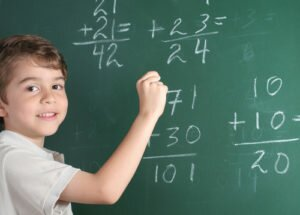Multiplication is an operation that requires you to add another number to itself a certain number of times as indicated in the multiplication equation.

When students first start learning the concept of multiplication, it is more simple as time goes on for kids to learn. Memorizing multiplication facts works for some students but not for all! Some students need to learn by using different models and representations. When students have a conceptual understanding of multiplication and realize that it is connected to the real world, they tend to perform better on assessments. If a child is only ever taught isolated facts or memorized facts, they risk the chance of not understanding the meaning behind the objects they are multiplying. Knowing a variety of ways to solve multiplication problems will allow a student to figure out which strategy works best for them.

## Pass The Times Tables Test!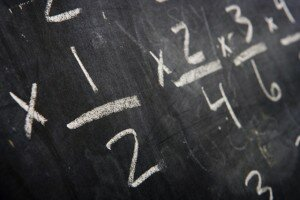Here’s a game that makes learning fun! Instead of giving your students yet another worksheet to complete, take those same problems, write them on index cards and turn it into a game. Practicing this way keeps your students focused, on-task and alert.

Preparation:

1)    Before class, create numbered index cards with the problems you want the students to solve (see figure 1). Create one card for each student in your class.

2)    Set up the classroom. I find that it is easiest to set the desks up in a circle so that students pass the cards clockwise and there is no confusion about who to pass the card to. Before the game, I also make all the students point to the person to whom they will pass their card.

3)    Prepare the recording sheet. It is extremely helpful to provide students with a numbered table to record their answers. I find it is beneficial to have students put a star on the number problem that they are starting with so they record their answers in the correct box. (See figure 2 for an example).

How to Play:

1)    Give each student a blank recording sheet. Then distribute the problems to solve. Each student is given a different index card to begin the game. Have the students put a star on the problem they will start with.

2)    Have the students solve the problem in front of them. Once they are done, the teacher says, “Pass” and all the students pass their card to the person next to them. Students then work on the problem on the card they just received, making sure they record it in the correct box.

3)    Continue this way until the students have completed each problem.

4)    Have the students provide the answer for the card they are left holding at the end of the game.

Notes:

1)    This game is not a timed activity, but most students find it more exciting when they have a set amount of time to finish. I tend to supervise the class and when it appears everyone is finished, I start counting down from 5.

2)    Enforce the rule that students are not permitted to pass cards until the teacher says, “Pass.” Otherwise, students end up with more than one card on their desks and end up passing the wrong card.

3)    I have used this game with every subject and grade level ever taught. It is a great way to practice vocabulary, review for an exam, assess student understanding, etc.

For more fun and interesting Learning Math Games, you can visit us here:
http://www.math-lessons.ca/activities/FractionsBoard5.html
http://www.math-lessons.ca/timestables/times-tables.html
http://www.math-lessons.ca/activities/FractionsBoard4.html

## Easter Egg Hunt 100 and Under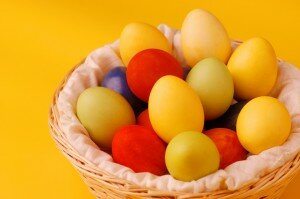Easter is the central feast and holiday in the Christian year representing Jesus’ Ascension.  It culminates the end of 40 days of fasting from the person’s choice.  Some people refrain from eating chocolate for 40 days until Easter weekend! Then they have an Easter Egg Hunt whereby one person hides chocolate eggs and the others have a fun treasure hunt to find the eggs.  For the Unity Spiritual Community, this year’s Easter’s theme is “Release Your Inner Splendor”. http://www.unity.org/publications/free-materials/lent-2012-release-your-inner-splendor

The following is a Fun Easter Egg Hunting Game for Practicing Addition, Subtraction, Multiplication and Division Under 100.  It can be played outdoors or indoors, depending on the weather and environment of the class.

Materials:

Organic Chocolate Eggs with foil covering

Marker

Pencil

Paper

Instructions:

Find a box of Organic Chocolate Easter Eggs.

Unwrap each egg but save the foil covering.

1. Make up a set of Math Problems to Solve, including addition, subtraction, multiplication and division.  At the end of this article, we have included a sample.

2. Wrap each Egg with a problem written on a small piece of paper, and then rewrap the eggs with the foil.  With a marker, number the eggs by groups.

3. Divide Learners into groups of 3, and Assign each group a number.  (In this example, there are 21 students. The class would be divided into 7 groups of 3, and there would be 7 groups of Math Problems).

4.Hide the eggs outdoors and/or indoors, weather permitting.

5. Each group has paper and pencil.  Each group hunts for eggs with their numbers and solves the math problems. As they find the eggs, each group respectively puts them in their basket. Each Learner Individually solves the problem, by first writing the problem on his/her paper and then following with the answer.

6. As each is finished, they bring their sheets to Teacher for checking. If they have any answers wrong, they must redo their answer. When all answers are correct, they can eat the chocolate, and help other groups.

Remember to recycle all the foil and paper wrappings!

http://kidsblogs.nationalgeographic.com/greenscene/2012/03/recycled-kisses.html

http://www.bayroberts.com/green/reduce.htm

Here are some more of our Fun Learning Math Games:

http://www.math-lessons.ca/activities/index.html

http://www.math-lessons.ca/review/math-review4.html

http://www.math-lessons.ca/timestables/times-tables.html

Sample Math Problems:

Group 1:

84 + 11 = 95

88 – 35 = 53

24 */* 3 = 8

8 x 6 = 48

14 */* 7 = 2

88 – 31 = 57

4  x 18 = 72

75 */* 3 = 25

25 – 5 = 20

Group 2:

88 + 12 = 100

25 x 3 = 75

18 */* 2 = 9

22  x  4 = 88

21 */* 3 = 7

55 –11 = 44

23 x 4 = 92

24 */* 12 = 2

45 + 33 = 78

Group 3:

88 – 3 = 85

13 x 4 = 52

100 */*25 = 4

44 x 2 = 88

13 – 1 = 12

44 */* 4 = 11

77 */* 11 = 7

3 x 9 = 27

52 – 23 = 29

Group 4:

44 – 11 = 33

23 x 4 = 92

14 */* 2 = 7

28 + 14 = 42

55 */* 11 = 5

88 – 13 = 75

34 – 17 = 17

22 x 4 = 88

14 + 17 = 31

Group 5:

14 + 5 = 19

33 – 12 = 21

22 x 5 = 77

89 – 17 = 72

34 +55 = 89

33 */* 3 = 11

22 x 4 = 88

100 */* 4 = 25

21 x 4 = 84

Group 6:

19 + 24 =43

47 – 17 =30

88 */* 11 = 8

24 x 3 = 72

90 */* 10 = 9

55 – 23 = 32

17 x 5 = 85

9 */* 3 = 3

100 – 25 = 75

Group 7:

18 */* 9 = 2

7 x 7 = 49

21 x 4 = 84

88 – 55 = 33

21 + 4 = 25

99 */* 3 = 33

22 x 1 = 22

35 – 8 = 27

77 – 22 = 55

Meteor Multiplication assists students in learning multiplication. This is a fun video game for learning elementary multiplication. Large meteors with multiplication problems in them move from all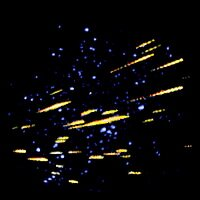around the screen toward a large star station in the center of the screen. Answers to the problems are placed in the center of the star station, the station gun is aimed at the approaching meteor,… and the gun is fired to disintegrate the meteor. If a meteor reaches the star station before disintegrated with the correct answer, the meteor hits the station and shatters. Answers are placed in the star station and gun moved and fired by designated keyboard keys. Hits and misses are recorded in the galaxy at the bottom of the screen. For a link to the game, you can go here:

And for one of our fun games for teaching multiplication, division, subtraction and addition, you can try our Quiz Master:

http://www.math-lessons.ca/review/math-review4.html

## Puzzle Pieces for Multiplication Tables

Almost everyone loves the mystery of solving puzzles. They give us a chance to use our critical thinking skills – and, give us a sense of accomplishment when we are able to see the final product. By putting together a picture, separated into pieces, you are showing the class that sometimes more than one answer is required in order to solve the problem. You will also be demonstrating the importance and the value of teamwork. This is a great activity to use when teaching the students. What is even better is that you get involved as well, so everyone is included in this fun game.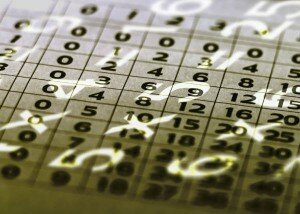Materials:

A 4×6 Poster or any size you desire

Construction Paper

Dark Marker

Regular Paper

## Learning Multiplication with Playing Cards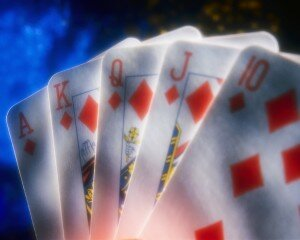This is a fun game for teachers to incorporate into their teaching lessons and it is fun because of the partial element of surprise. Kids love surprises and the best part of this game is that the students will have even more fun learning because they won’t even realize they are in class. This will make learning how to multiply even less of a hassle for teachers.

What you will need:

Deck of cards per 3 players at a time- average class size 30-35 (12 decks)

## Multiplication MastersYou can teach students a fun activity incorporating multiplication times-tables with this inquisitive game that includes the concept of a point system. Who doesn’t love a little competition? Just about everyone, including children. With that in mind, how about teaching your students a little about intrinsic and extrinsic motivation as well?

## Sarah Jane learn the EZ Times Table©

http://www.RightBrainMath.com/ A second grade student learns to multiply and create the multiplication table in one hour in this patent pending system. She also learns division, addition, subtraction, factors, squares, fractions, tables and graphing. This shows how to teach using the book, EZ Times Table. It was written by Tom Biesanz and illustrated by Jakob Marsh.There are also playful patterns for creating the Ones, Twos, Threes, Fours, Sixes (five different ways) Sevens, Eights, Nines, and even the whole times table on a blank table. Kids, Teachers, Parents, and schools will benefit from this fun way to approach the times table that creates a positive attitude about math. The editor of Curriculum Review magazine says, “I like the book [EZ Times Table] because it is user-friendly and can be utilized with a variety of grade-school children.” See the book EZ Times Table available on Amazon.com and on the website http://RightBrainMath.com.

Original post:
Sarah Jane learn the EZ Times Table©

## Times Tables – recent posts

Times tables?

Knowing the times tables is just a shortcut. if you understand the math, you don't need to know the tables as you can always work out the answer. But you must know one or the other, lest you end up unable to manage your finances and people steal